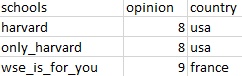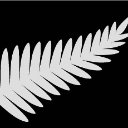## Error in macro statement

Hi,

I would like to find only these cases in which "schools" variable has at least one value from "place" variable. Then I want to also add to these cases the right values of "opinion" and "country" variables. Using datasets which I created in SAS (code below) the results should look like:That's my code:

``````data aaa;
input  schools: \$50.;
datalines;
harvard
only_harvard
cheese_cake_pizza
wse_is_for_you
;
proc print data=aaa noobs;
run;
data bbb;
input  place: \$10. opinion: 2. country: \$10.;
datalines;
harvard 8 usa
oxford 8 uk
wse 9 france
;
%macro xxx(data_out=);
%let listopinion=;
proc sql noprint;
select opinion
into :listopinion separated by "!"
from bbb
;
run;
quit;
%let listcountry=;
proc sql noprint;
select country
into :listcountry separated by "!"
from bbb
;
run;
quit;
%let listplace=;
proc sql noprint;
select place
into :listplace separated by "!"
from bbb
;
run;
quit;
data &data_out;
set
%let i_1 =1;
%let i_2 =1;
%let i_3 =1;
%do %until (%qscan(&listcountry,&i_3,'!') = %str());
%do %until (%qscan(&listopinion,&i_2,'!') = %str());
%do %until (%qscan(&listplace,&i_1,'!') = %str());
%let zm1=%scan(&listplace,&i_1,'!');
find(schools,"&zm1") >0
%let i_1=%eval(&i_1+1);
%end;
%let zm2=%scan(&listopinion,&i_2,'!');
%let i_2=%eval(&i_2+1);
%end;
%let zm3=%scan(&listcountry,&i_3,'!');
%let i_3=%eval(&i_3+1);
%end;
run;
keep school &zm1 &zm2 &zm3;
%mend;

%xxx(data_out=ddd);``` ```ChrisNZ
Tourmaline | Level 20

## Re: Error in macro statement

Something like this?

``````proc sql;
select SCHOOLS, OPINION, COUNTRY
from AAA
,BBB
where index(SCHOOLS, PLACE, 't' );``````
Discussion stats